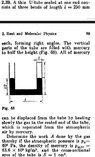# Krotov problem: how to write Energy conservation for this fluid?

• ermia
ermia
Homework Statement
I uploaded the problem. when I want to write the potential energy of the fluid in the initial and final state I don't find the right answer. I know the rest of the problem.
Relevant Equations
$$U=\rho g h A h_{cm}$$
I wrote some potentials but they were wrong. I used the cm of all fluid parts and I used the radius which is $$\sqrt S/ \pi$$ .

#### Attachments

•Apt.jpg
40.7 KB · Views: 16

ermia
That equation for radius has a "type" error.
$$r =\sqrt \frac{S}{\pi}$$

Homework Helper
Gold Member
2022 Award
Per forum rules, please post your working. Otherwise we have no way to know where you are going wrong.

Homework Helper
Gold Member
Could you explain how is that radius, which is not shown as data in the problem, relevant?

•